Processing ......FreeComputerBooks.com Links to Free Computer, Mathematics, Technical Books all over the World

Differential Equations and Dynamical Systems
Related Book Categories:
•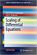Scaling of Differential Equationsn (Hans Langtangen, et al.)

Scaling is a classical topic in applied mathematics, but here strongly connected to numerical simulations. The book contains a wide range of examples, of differing complexity, from many different scientific fields.

•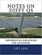Notes on Diffy Qs: Differential Equations for Engineers (Jiri Lebl)

An introductory course on differential equations aimed at engineers. The book covers first order ODEs, higher order linear ODEs, systems of ODEs, Fourier series and PDEs, eigenvalue problems, the Laplace transform, and power series methods.

•Finite Difference Computing with PDEs: A Software Approach

This easy-to-read book introduces the basics of solving partial differential equations by means of finite difference methods. Unlike many of the traditional academic works on the topic, this book was written for practitioners.

•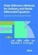Finite Difference Methods for Differential Equations

This book introduces Finite Difference Methods for differential equations and discusses the similarities and differences between algorithm design and stability analysis for different types of equations.

•Solving PDEs in Python: The FEniCS Tutorial I (H. Langtangen)

This book offers a concise and gentle introduction to finite element programming in Python based on the popular FEniCS software library. Using a series of examples, it guides readers through the essential steps to quickly solving a PDE in FEniCS.

•A Friendly Introduction to Differential Equations (M. Kaabar)

Very useful for college students who studied Calculus II, and other students who want to review some concepts of differential equations before studying courses such as partial differential equations, applied mathematics, and electric circuits II.

•Elementary Differential Equations (William F. Trench)

This text has been written in clear and accurate language that students can read and comprehend. The author has minimized the number of explicitly state theorems and definitions, in favor of dealing with concepts in a more conversational manner.

•Elementary Differential Equations with Boundary Value Problems

Written in a clear and accurate language that students can understand, this book minimizes the number of explicitly stated theorems and definitions, includes a thorough treatment of boundary-value problems and partial differential equations.

•Stochastic Differential Equations: Models and Numerics

The goal of this book is to give useful understanding for solving problems formulated by stochastic differential equations models in science, engineering and mathematical finance. Typically, these problems require numerical methods to obtain a solution.

•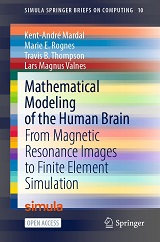Mathematical Modeling of the Human Brain

The book bridges common tools in medical imaging and neuroscience with the numerical solution of brain modelling PDEs, covers the basics of magnetic resonance imaging and quickly proceed to generating first FEniCS brain meshes from T1-weighted images.

•Linear Partial Differential Equations and Fourier Theory

This highly visual introductory textbook presents an in-depth treatment suitable for undergraduates in mathematics and physics, gradually introducing abstraction while always keeping the link to physical motivation.

•Complex and Adaptive Dynamical Systems: A Primer (Claudius Gros)

Discover a wide range of findings in quantitative complex system science that help us make sense of our complex world. Written at an introductory level, the book provides an accessible entry into this fascinating and vitally important subject.

•Asymptotically Almost Periodic Solutions of Differential Equations

This book is dedicated to the study of asymptotically stable in the sense of Poisson (in particular, asymptotically almost periodic) motions of dynamical systems and solutions of differential equations.

•Solvability of Nonlinear Singular Problems for ODEs

This book is devoted to singular boundary value problems for ordinary differential equations. It presents existence theory for a variety of problems having unbounded nonlinearities in regions where their solutions are searched for.

•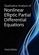Qualitative Analysis of Nonlinear Elliptic PDEs

The book provides a comprehensive introduction to the mathematical theory of nonlinear problems described by elliptic partial differential equations. Much of the modern theory of such equations is based on the calculus of variations and functional analysis.

•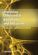Impulsive Differential Equations and Inclusions (M. Benchohra)

This book is devoted to impulsive differential equations and inclusions. Initial and boundary value problems for both impulsive differential equations and inclusions, as well as for each of impulsive functional differential equations or inclusions, and neutral functional differential equations, are studied.

•Discrete Oscillation Theory (Ravi P. Agarwal, et al)

This book is devoted to a rapidly developing branch of the qualitative theory of difference equations with or without delays. It presents the theory of oscillation of difference equations, exhibiting classical as well as very recent results in that area.

•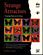Strange Attractors: Creating Patterns in Chaos (Julien C. Sprott)

Describes a simple method for generating an endless succession of beautiful fractal patterns by iterating simple maps and ordinary differential equations with coefficients chosen automatically by the computer. Each pattern produces a corresponding piece of fractal music.

•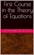First Course in the Theory of Equations (Leonard Dickson)

The theory of equations is not only a necessity in the subsequent mathematical courses and their applications, but furnishes an illuminating sequel to geometry, algebra and analytic geometry. A useful supplement to differential calculus.

•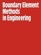Boundary Element Methods in Engineering and Sciences

This book is designed to provide readers with a comprehensive and up-to-date account of the Boundary Element Methods (BEM) and its application to problems in engineering and science, with historical development, basic theory, derivation and examples.

•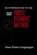Introduction to Finite Elements Methods (Hans P. Langtangen)

This book is aimed at presenting the theory and practice of Finite Element Method (FEM) in a manner which makes it is easy to learn the concepts, analysis, and methodology of FEM through simple derivations and worked out examples in interdisciplinary areas.

•Finite Element Analysis (David Moratal)

This book provides a bird's eye view on this very broad matter through original and innovative research studies exhibiting various investigation directions. You will gain a better understanding of where Finite Element Analysis stands today.

•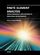Finite Element Analysis: Biomedical Apps to Industrial

This book presents represents a numerical technique for finding approximate solutions to partial differential equations as well as integral equations, permitting the numerical analysis of complex structures based on their material properties.

•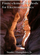Finite-element Methods for Electromagnetics (Stanley Humphries)

This book covers a broad range of practical applications involving electric and magnetic fields. The text emphasizes finite-element techniques to solve real-world problems in research and industry.

•Finite Difference Computing with PDEs: A Software Approach

This easy-to-read book introduces the basics of solving partial differential equations by means of finite difference methods. Unlike many of the traditional academic works on the topic, this book was written for practitioners.

Book Categories
 :All CategoriesRecent BooksMiscellaneous BooksComputer LanguagesComputer ScienceData Science/DatabasesElectrical EngineeringJava and Java EE (J2EE)Linux and UnixMathematicsMicrosoft and .NETMobile ComputingNetworking and CommunicationsSoftware EngineeringSpecial TopicsWeb Programming
Other Categories